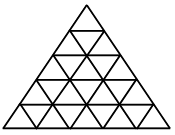Find the number of triangles in the given fig?

# Find the number of triangles in the given fig?1. A

48

2. B

44

3. C

46

4. D

52

Fill Out the Form for Expert Academic Guidance!l

+91

Live ClassesBooksTest SeriesSelf Learning

Verify OTP Code (required)

### Solution:

No. of triangle in each base = 5

So$=\frac{\left[n\left(n+2\right)\left(2n+1\right)\right]-1}{8}$

$⇒\frac{\left(5×7×11\right)-1}{8}$

$⇒\frac{384}{8}=48$+91

Live ClassesBooksTest SeriesSelf Learning

Verify OTP Code (required)